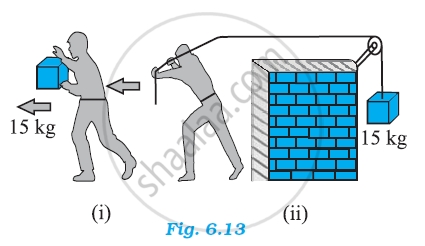# In Figure (I) the Man Walks 2 M Carrying a Mass of 15 Kg on His Hands. in Figure (Ii), He Walks the Same Distance Pulling the Rope Behind Him. the Rope Goes Over a Pulley, and a Mass of 15 Kg Hangs at Its Other End. in Which Case is the Work Done Greater - Physics

In Figure (i) the man walks 2 m carrying a mass of 15 kg on his hands. In Figure (ii), he walks the same distance pulling the rope behind him. The rope goes over a pulley, and a mass of 15 kg hangs at its other end. In which case is the work done greater ?#### Solution 1

In the second case

Case (i)

Mass, m = 15 kg

Displacement, s = 2 m

Work done W = Fs cos theta

Where theta = Angle between force and displacement.

=mgscostheta = 15 xx 2 xx 9.8 cos 90^theta

= 0              (∵ cos 90^@ = 0)

Case (ii)

Mass, m = 15 kg

Displacement, s = 2 m

Here, the direction of the force applied on the rope and the direction of the displacement of the rope are same.

Therefore, the angle between them, θ = 0°

Since cos 0° = 1

Work done, W = Fs cosθ = mgs

= 15 × 9.8 × 2 = 294 J

Hence, more work is done in the second case.

#### Solution 2

In Fig. (i), force is applied on the mass, by the man in vertically upward direction but distance is moved along the horizontal.

θ = 90°. W = Fs cos 90° = zero

In Fig. (ii), force is applied along the horizontal and the distance moved is also along the horizontal.

Therefore, θ = 0°.

W = Fs cos θ = mg x s cos 0°

W = 15 x 9.8 x 2 x 1 = 294 joule.

Thus, work done in (ii) case is greater.

Concept: Concept of Work
Is there an error in this question or solution?

#### APPEARS IN

NCERT Class 11 Physics
Chapter 6 Work, Energy and Power
Q 5.4 | Page 135